# Curve Conveying

The different diameters over the length the tapered roller i.e. small one end and large the other end, provides a linear velocity to ensure smooth conveying of goods around the curve.
The roller axis is installed at a defined angle θ / 2 (θ = 3.6o).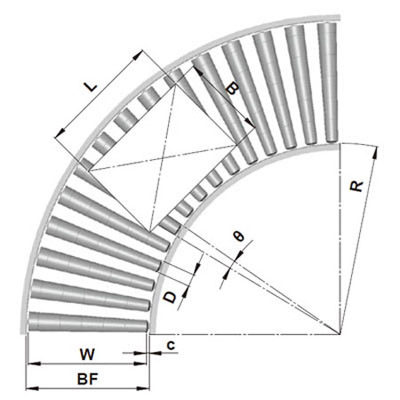Roller Pitch
The design of roller pitch should follow the principle of minimum 3 rollers to support the goods at any moment.
For 2650 series poly-vee tapered rollers the recommended pitch of poly-vee pulleys is 73.7mm.
The angle between tapered rollers should not exceed 5o.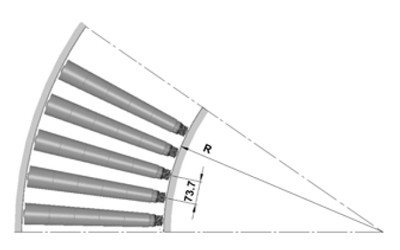In theory, the geometric extension line of the tapered roller should join with the centre of the radius of the curve frame. By using this method, you can achieve the ideal curve for conveying. It may be calculated using the formula below: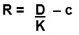Where:
D –  diameter of the smaller head of the taper roller.
K –  conical degree (the conical degree is expressed by fraction, e.g.: 1/16, 1/30, it’s reduction formula is K = 2 x tan θ / 2.
c –   the space between the tapered roller’s smaller head and the inner side of the frame.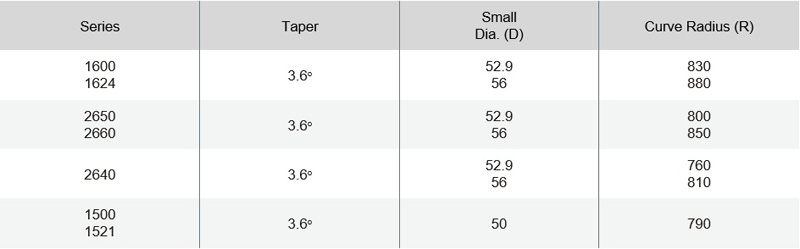Calculating Roller Length
For straight conveying, generally there is no need to consider the length of the goods but for curved conveying, the length and width of goods and the curve radius are all influencing factors. It may be calculated using the formula below: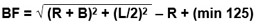Where:

BF –  frame inner width.